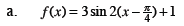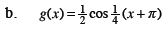Home > CCA2 > Chapter 9 > Lesson 9.2.2 > Problem9-69

9-69.
1. Graph at least two cycles of the following functions. Homework Help ✎

1. f(x) = 3 sin 2(x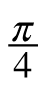) + 1

2. g(x) =cos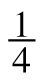(x + π)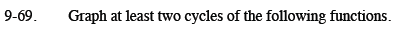Identify the amplitude, period, horizontal shift, and vertical shift for each function.

Review the Math Notes box in Lesson 7.2.4 for help identifying the important parts of the function.